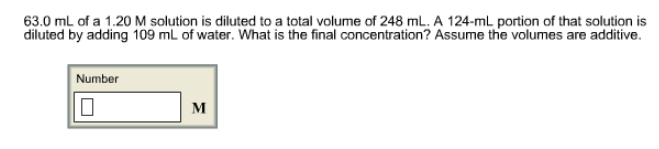# Problem: 63.0 mL of a 1.20 M solution is diluted to a total volume of 248 mL. A 124-mL portion of that solution is diluted by adding 109 mL of water. What is the final concentration? Assume the volumes are additive.

###### FREE Expert Solution
86% (70 ratings)###### Problem Details

63.0 mL of a 1.20 M solution is diluted to a total volume of 248 mL. A 124-mL portion of that solution is diluted by adding 109 mL of water. What is the final concentration? Assume the volumes are additive.